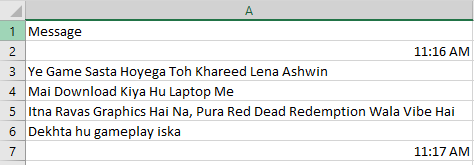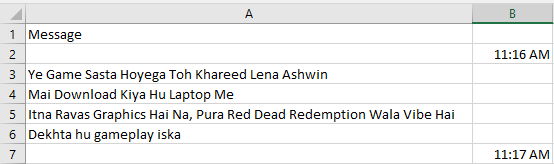# How to print the string in next row if it ends with time

I have a table like this in Excel :What I need to do is if the row data ends with time I need to move that row into the next column

Required output :2. Then put a If activity inside For each with condition as below,
`System.Text.RegularExpressions.Regex.IsMatch(row("Message").ToString.trim,"[0-9]{2}:[0-9]{2}")`
above expression gives you Boolean type.
3. And in then part of If activity use assign activity and write as below,
`row("Your Second Column Name") = row("Message").ToString`

Hope this might help you2 Likes

@Ishan_Shelke

Try as below

1. Use a read range activity and read data into datatable dt…
2. If second column is missing then use add data column activity to add a column
3. Now use for each row in datatable activity on dt
4. Inside loop use if condition with `System.Text.RegularExpressions.Regex.IsMatch(currentrow(0).ToString,"\d{2}:\d{2} [A-Z]{2}")`
5. On the then side use assign with `currentrow(1) = currentrow(0).ToString`

Hope this helps

Cheers

build a datatable where one colmn is for message another for time.
for each row first_dt MatchFound go and add datarow to outputDt-{Currentrow(0).tostring,Currentrow(1).tostring.trim}